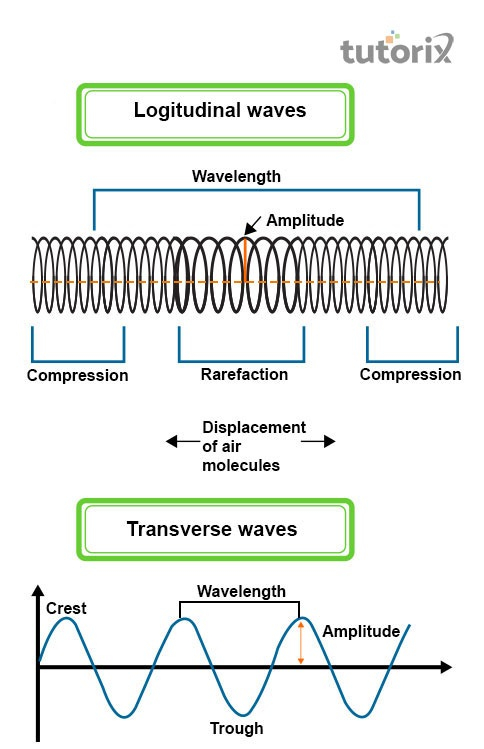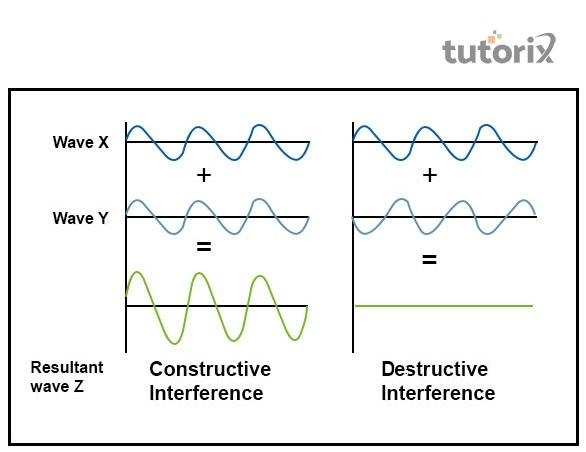# Travelling Wave

## Introduction

Waves are determined as the disturbance in the medium through which it is travelling and transferring energy and momentum without any net motion of that medium. If the energy travels from one place to another, it is transported through these travel waves. The standing waves never transport energy like travelling waves. In another word, these travel waves can also be denoted as the wave in which positions of minimum and maximum amplitude are travelling along with the medium. The equation that can describe the travelling waves is y(x,t) = (0.003) cos(20 x + 200 t ). Here, x and y are measured in meters and t in seconds.

## What is Wave?

The wave is basically determined as the disturbance of medium that is carrying a certain amount of energy without any movement of particles. During the travelling of waves, only energy is transferred. No matter is transferred by the travel waves. Waves move through a medium and as the medium moves forth and back repeatedly, and then returns to its original position waves are generated.Figure 1: Longitudinal waves Vs Transverse waves

The two main types of waves are longitudinal waves and transverse waves. If the material in a medium moves in the same dimension with respect to the direction of wave movement, then this phenomenon is denoted as the longitudinal waves (Salehi et al. 2018). If the waves in the medium move at their right angle with respect to the direction of wave movement, then this phenomenon is denoted as the transverse waves.

## Travelling Wave: Explanation

The disturbance in the medium moves gradually outwards away from its source in all directions with respect to the phenomenon of travelling waves. The information mainly travels in the form of waves from one place to the others. The major examples of travelling waves are electromagnetic radiation coming out from mobile phones, the sound coming to the ears, and light rays falling on eyes, which are then transported in form of travel waves. The visual example of travel waves is the waves that are formed on the water surface when a stone is dropped into a pond.

On jumping on a trampoline, the push that is created downward at one of its points makes the material moves slightly downward too. On the other side, if the disturbance is formed outward, then the point where the feet will first hit the trampoline will be found moving outward as the tension force in the trampoline (Gao et al. 2017). This tension force will also make the surrounding particles or area of the trampoline move outwards. The down and up motion of disturbance created on trampoline takes the shape of travel waves.

## Types of Travelling Waves

There are several travelling waves that have distinct characteristics as mentioned below.

• Continuous Waves: These types of waves have waveforms of constant frequency and amplitude.

• Transverse Waves: Here, the particle motion remains perpendicular to the propagation direction of the travel waves (Ding et al. 2017).

• Pulse Waves: These waves have only 1 crest and 1 disturbance travelling along with transmission medium.

• Longitudinal Waves: Here, the particle motion remains in the same direction as propagation of travelling waves.

## Destructive and constructive Waves

In the context of travel waves, it can be stated that this is the phenomenon of 2 waves superimpose and form a resultant wave of greater, equal or lower amplitude, which is denoted as the wave interference. The 2 main types of wave interference are constructive interference and destructive interference.

Constructive Interference: This kind of wave interference mainly happens when the representing peaks or lines, cross over one another. In simple words, it can be mentioned that when 2 waves are in one phase they will interfere constructively.Figure 2: Wave Interference

Destructive Interference: This type of wave interference happens when 2 waves move out of phase completely and in this situation, the representing peak will lie at the middle point of 2 waves. It can be further illustrated when 2 waves are out-phase by π radians or 180° they will interfere destructively cancelling out one another.

## Conclusion

The high point of travelling waves is determined as the crests and the lower points are denoted as troughs. The distance between 2 adjacent troughs and 2 adjacent creates is called the wavelength and can be denoted by ƛ. In the context of the maximum distance of disturbance in the medium of travel wave from its middle-point to either bottom of trough or top of crests is determined as the amplitude. The time that travels of 1 complete vibration is denoted as period and the number of vibrations that the travelling waves undergo in 1 second is denoted as its frequency. The speed of a travelling wave can be written as V= ƛ f.

## FAQs

Q1. What do you mean by pulse wave?

Ans. The travelling waves that comprise only 1 disturbance are denoted as the pulse waves. Here the waves also comprise 1 crest travelling along with the transmission medium.

Q2. What are longitudinal waves?

Ans. The disturbance that occurs when an individual jumps on a trampoline is also referred to as the form of travelling waves. In the case of longitudinal waves, the particles’ motion is in the same direction as propagation of the travelling waves.

Q3. How the travel wave is generated?

Ans. Travelling waves is also considered as the wavetrain is the periodic function having 1-dimensional space move with content speed. This wavetrain is a type of spatiotemporal oscillation which is the periodic function of both time and space.

Q4. What is the function of travelling waves in a power system?

Ans. On the line of transmission the travel waves is the current or voltage waves that propagate from source end to load end at the time of transient condition. The waves here travel along a line with velocity equivalent to the light velocity when the line losses is neglected.

Updated on: 13-Oct-2022

290 Views